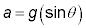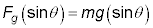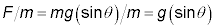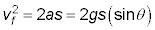##### Physics I Workbook For Dummies with Online PracticeWhen objects slide (frictionlessly) down a ramp, they’re acted on by a force, which means that they’re accelerated and therefore their speed changes. The equation to use in physics problems like these is

vf2vo2 = 2as

Finding the object’s final speed under these circumstances is easy when you remember thats is the length of the ramp, and vo is usually 0.

## Sample question

1. Say you have a block of ice on a ramp at 20 degrees, and it slides down a ramp of 5.0 meters. What is its final speed at the bottom of the ramp?

The correct answer is 5.8 m/s.

1. The force along the ramp is2. The acceleration of the ice is3. Use the equationPlug in the numbers: vf2 = 34, which means vf = 5.8 m/s.

## Practice questions

1. Starting from rest, you go down a 100 m ski jump of 60 degrees. What is your speed at takeoff?

2. You’re heading down a toboggan run of 1 km at an angle of 18 degrees. What is your final speed?

3. You have a block of ice on a ramp with an angle of 23 degrees when it slips away from you. What is its speed at the bottom of the 6.0 m ramp?

4. A cart starts at the top of a 50-m slope at an angle 38 degrees. What is the cart’s speed at the bottom?

Following are answers to the practice questions:

1. 40 m/s

1. The force along the ramp is3. Use the equation4. Solving for vf = 41 m/s. Round to 40 m/s to account for significant figures.

2. 80 m/s

1. The force along the ramp is3. Use the equation4. Solving for vf = 78 m/s. Round to 80 m/s to account for significant figures.

3. 6.8 m/s

1. The force along the ramp is2. The block of ice’s acceleration is3. Use the equation4. Solving for vf = 6.8 m/s.

4. 25 m/s

1. The force along the ramp is2. The cart’s acceleration is3. Use the equation4. Solving for vf = 25 m/s.